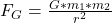## Now you know one way to determine the mass of objects which are being orbited, including black holes, but how do you think the mass of Mercu

Question

Now you know one way to determine the mass of objects which are being orbited, including black holes, but how do you think the mass of Mercury and Venus has been determined since they do not have natural satellites in orbit? Give a detailed process you could use to determine an experimental mass for these planets.

in progress 0
3 months 2021-08-15T07:58:12+00:00 1 Answers 6 views 0

See the explanation below

Explanation:

In order to calculate the mass of these planets, the law of universal gravitation proposed by Isaac Newton must be used. If we do not have satellites orbiting around the respective planet.

We should use the mass of a satellite known as the planet Earth, then knowing the gravitational force between the Earth and venus for a known distance we can find the mass of venuswhere :

FG = Gravitational force [N]

G = universal gravitation constant = 6.67*10^(-11) [N*m^2/kg^2]

m1 = mass of venus [kg]

m2 = mass of the earth [kg]

r = distance between the planets [m]

We can do the same to find the mass of Mercury.